VLOOKUP Column Index Number: What is It and How to Input It Correctly? - Compute Expert

# VLOOKUP Column Index Number: What is It and How to Input It Correctly?

Home >> Excel Tutorials from Compute Expert >> Excel Tips and Trick >> VLOOKUP Column Index Number: What is It and How to Input It Correctly?

From this tutorial, you will learn completely about the VLOOKUP column index number.

When we use the VLOOKUP function in excel, one of the inputs that we must give to VLOOKUP is the column index number. However, some of us might still not understand this input and what is the right number we should give. This tutorial is here to help you understand completely about the input so you can use the VLOOKUP function in excel more optimally.

Want to understand better about the VLOOKUP column index number input? Let’s get into it right away.

Disclaimer: This post may contain affiliate links from which we earn commission from qualifying purchases/actions at no additional cost for you. Learn more

## VLOOKUP Brief Description

As you may already know, VLOOKUP is a function we can use to find data vertically in excel. VLOOKUP does the data finding process by searching for the reference value we input into it on the most left column of our reference cell range to get the position and the value of the data we want to find.

In general, we write a VLOOKUP formula like this in excel.

= VLOOKUP ( reference_value , reference_cell_range , column_index_number , [ search_mode ] )

Here is a brief description of each of the VLOOKUP inputs:
• reference_value: the value that becomes the reference for VLOOKUP to find the data we want it to find. VLOOKUP will look up the reference value row position in the most left column of our reference cell range to get our desired data in the column that we determine
• reference_cell_range: the cell range where VLOOKUP will perform its lookup operation. The cell range is usually in the form of a data table
• column_index_number: the index of the column in the reference cell range from where we want to find and get our data
• [ search_mode ]: an optional input. Determines whether we want to find an exact match only for our reference value (FALSE) or an approximate match is okay too (TRUE). The default, if we don’t give input here, is TRUE or an approximate match for the reference value is okay

## What is the VLOOKUP Column Index Number?

The VLOOKUP column index number is the third required input in a VLOOKUP formula that determines in which column our VLOOKUP is going to get its result (the data we want to find with the VLOOKUP). The input here, as you may already suspect, must be a number and it cannot be more than the number of columns that the reference cell range in our VLOOKUP has.

## Why is the VLOOKUP Column Index Number Important?

The column number index that we input to our VLOOKUP is important because it determines the result we get from our VLOOKUP. That is because this input tells VLOOKUP from which column in the reference cell range that it should get its result.

Wrong input here can cause VLOOKUP to look for our data in the wrong column and, thus, make us get the wrong VLOOKUP result. It might even cause our VLOOKUP to produce an error if we give a non-number input or a number input that is more than the number of columns that our reference cell range has.

## How to Determine the VLOOKUP Column Index Number of the Column We Want to Input?

It is not that difficult to determine the column index number we should input into our VLOOKUP. Just count the order of the column from where we want to get our VLOOKUP result from left to right from the first column of our reference cell range. This column order is the number that we should input as the third input of our VLOOKUP.

For example, take a look at the screenshot below.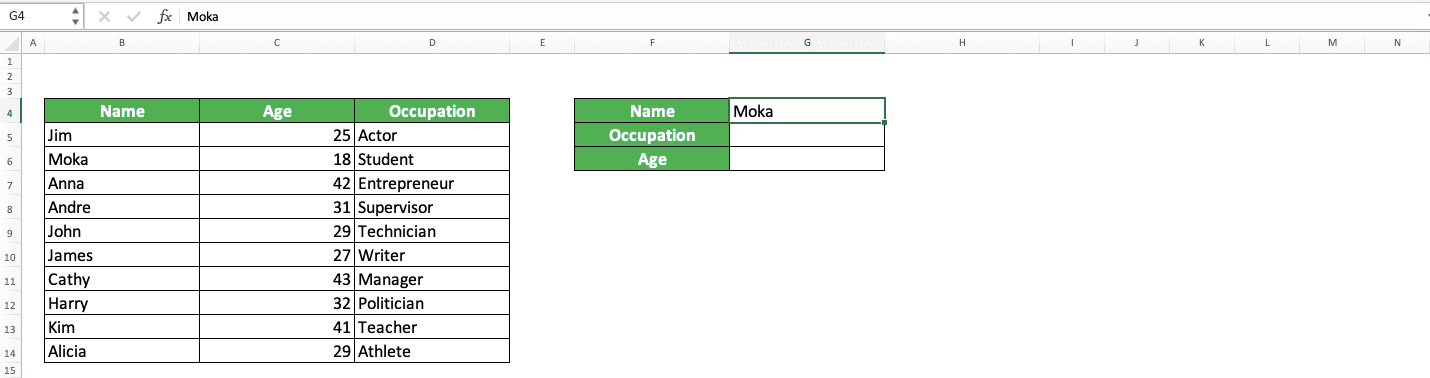If we want to get Moka’s occupation (or anyone else) from the table on the left, what column index number should we input into our VLOOKUP? Well, we can know the “Occupation” column index number on the table by counting its order from the first column of the cell range that we will input to our VLOOKUP.The cell range we will input into our VLOOKUP is obviously the cell range of the table on the left. As the first column of that table is the “Name” column, we should just count the order of the “Occupation” column from that column from left to right. Thus, the column index number for this “Occupation” column here is 3.

We use this 3 as our third VLOOKUP input and we will get the occupation data we want!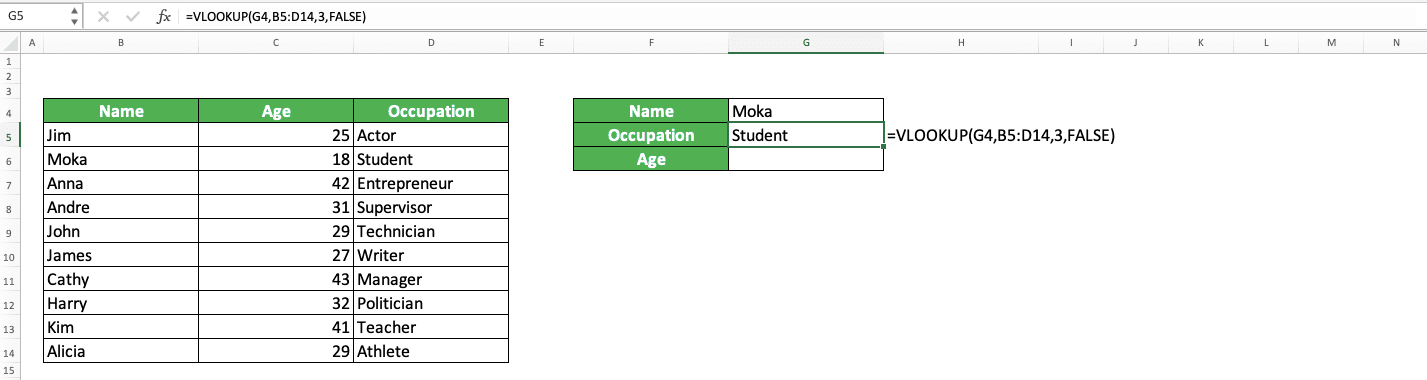What about if we want the age instead? For that, we just need to do the same to determine the column index number of the “Age” column from where we will take this age data.

As you can see in the previous second screenshot, the order of the “Age” column here from the “Name” column is 2. So, we input 2 as our third VLOOKUP input to get the age data.Quite simple, isn’t it? So, next time you have to input the column index number of your VLOOKUP, you don’t have to be confused. Just count the order of the column where you want to get your VLOOKUP result from left to right from the first column of your reference cell range.

By doing that, you should get the correct column index number input you need to get the correct result from your VLOOKUP.

## How do We Input the VLOOKUP Column Index Number the Right Way?

To input a column index number into your VLOOKUP formula, just type the number as the third input of your VLOOKUP. Make sure you input the right column index number or you will get a wrong/error VLOOKUP result.

You can also input the number by inputting a cell coordinate that contains the column number index you want. If you input the number this way, it will be easier for you to change it in case you want to change the column from where you get your VLOOKUP result (just need to change the number in the referred cell).

## Automatic VLOOKUP Column Index Number: VLOOKUP COLUMN

Got a table with many columns that you use as your VLOOKUP reference cell range and you don’t want the trouble of counting the correct column number index for your VLOOKUP? You can use the COLUMN function to help you with that problem.

Here is the general writing format of the VLOOKUP and COLUMN combination that makes you get your column index number automatically.

= VLOOKUP ( reference_value , reference_cell_range , COLUMN ( result_column_cell_coordinate ) - COLUMN ( first_column_cell_coordinate ) + 1 , [ search_mode ] )

Input two COLUMN formulas as your VLOOKUP column number index input with the format as written above.

COLUMN helps us get the column number of the cell coordinate we input into it. By subtracting the column number of the column where we want to get our VLOOKUP result with the column number of the first column of our reference cell range and adding the subtraction result with 1, we should get the correct column number index input for our VLOOKUP.

For an implementation example of the VLOOKUP COLUMN formula, take a look at the screenshot below. We use the same data we used in the previous example here.As you can see, we can get the same VLOOKUP results as before by using the VLOOKUP and COLUMN combination formula. Moreover, we don’t have to input the column index number directly here. We just need to use our COLUMN formulas to give us our correct column index number input!

## Flexible VLOOKUP Column Index Number: VLOOKUP MATCH

Want a flexible column index number input in your VLOOKUP where you can change your VLOOKUP result according to the data variable you currently want to find? You can do that by combining your VLOOKUP with MATCH.

Here is the general writing format of the two functions’ combination for that purpose.

= VLOOKUP ( reference_value , reference_cell_range , MATCH ( data_variable_cell_coordinate , column_header_row_exclude_first_column , 0 ) + 1 , [ search_mode ] )

We use MATCH in our VLOOKUP third input to let us control the column index number more flexibly.

In the MATCH formula, we input the cell coordinate that contains the data variable we currently want to find with our VLOOKUP and the column header row of our VLOOKUP reference cell range, which should contain all of our data variable names in the reference cell range, excluding the first column header (because the VLOOKUP result we want isn’t the reference value, which we can get from our VLOOKUP if we include the first column header). We add 1 to the MATCH result so we can get the correct column index number input for our VLOOKUP.

By doing this formula writing, we can just change the value of our data variable cell with another data variable available in the reference cell range and our VLOOKUP will adapt its result accordingly!

Additionally, it will become easier for you if you add a simple dropdown in the data variable cell which lists all of your data variable names. That way, you can be sure that the data variable name you input into your MATCH formula is the correct one.

To better understand the VLOOKUP MATCH combination to make your VLOOKUP column index number much more flexible, take a look at the screenshot below for its implementation example.As you can see, we can get the VLOOKUP result we want by combining VLOOKUP and MATCH functions in our formula. The formula writing format is as we have discussed before.

When we change the value of the data variable cell referred to in our MATCH formula, our VLOOKUP result changes accordingly.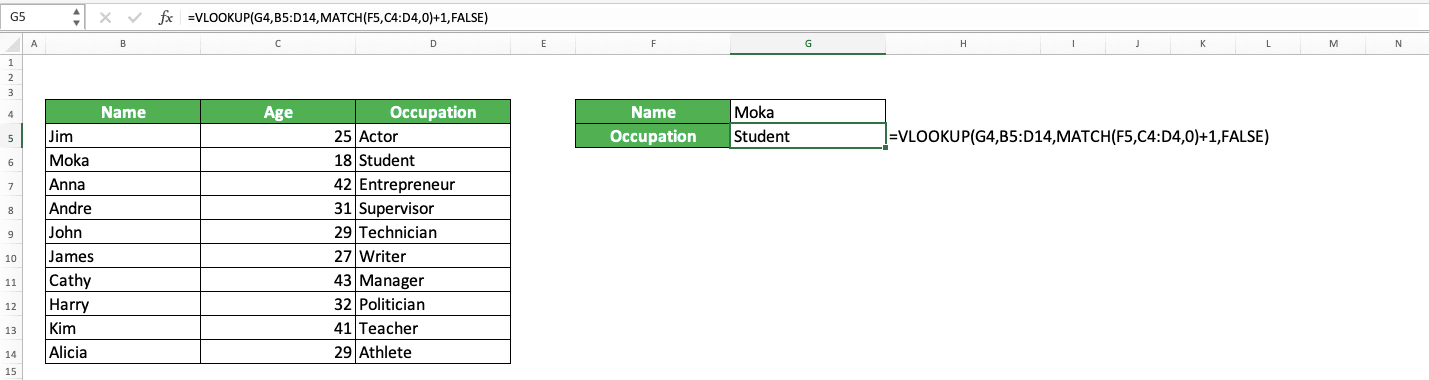Need to know how to create the dropdown in the data variable cell? The steps are simple.

First of all, select the cell that you want to refer to as your MATCH data variable cell. Then, go to the Data tab in your ribbon and click on the Data Validation button there.In the dialog box that shows up, in its Settings tab, click on the Allow dropdown.Choose List from the dropdown list.Then, click the button in the Source text box that shows up.Click and drag on the entire column headers of your VLOOKUP reference cell range, excluding the first column header. After their cell range coordinate is inputted, click on the button on the right side of your text box.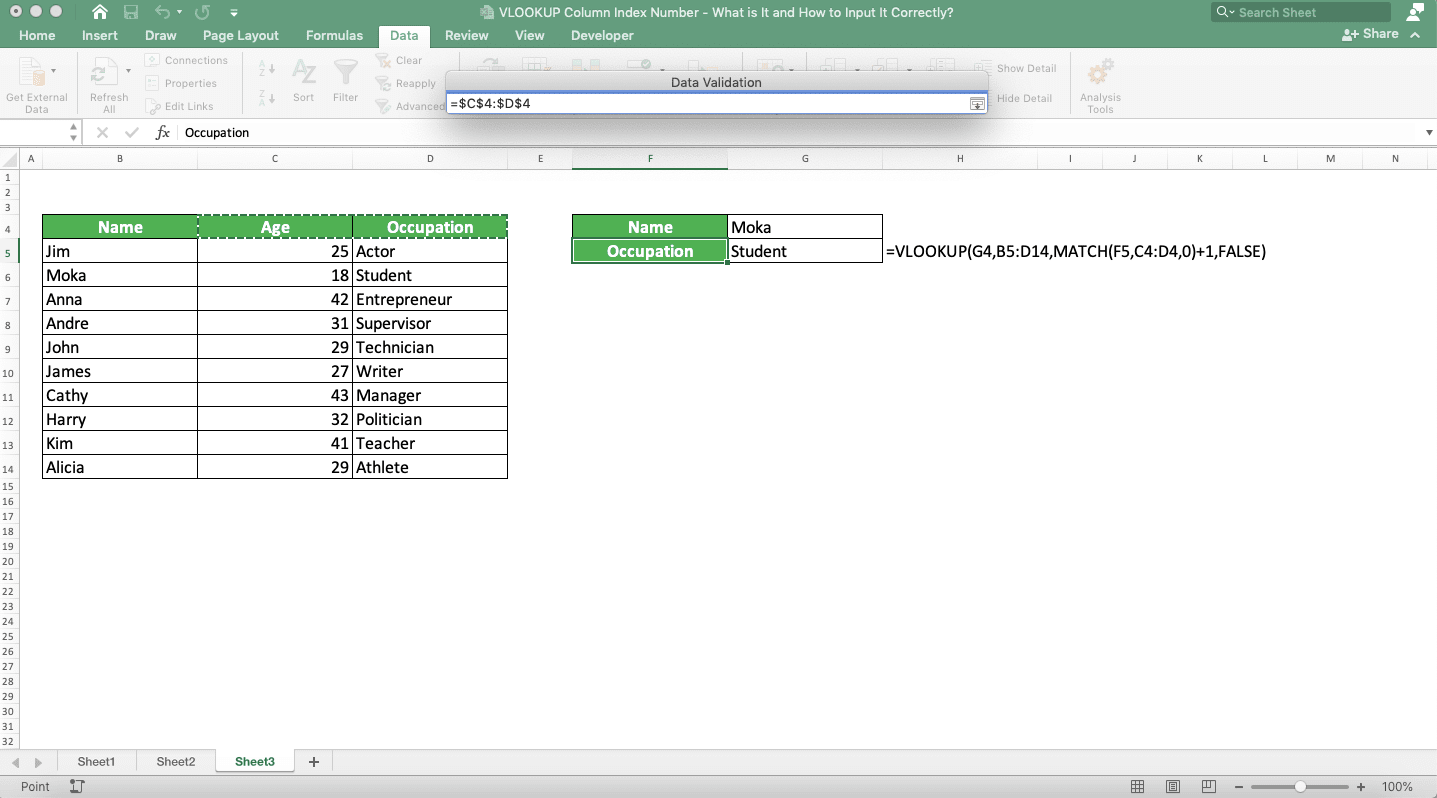Click OK.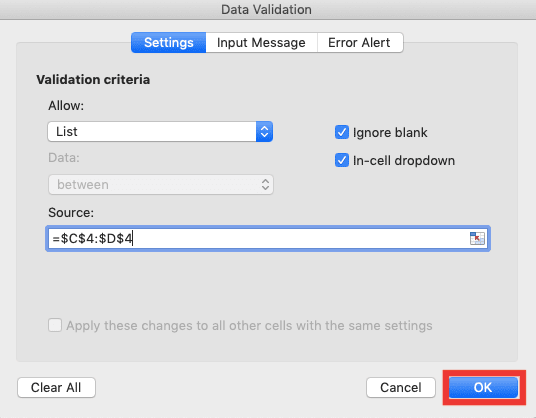That is done! Now, you can choose the data variable you want in your MATCH data variable cell from a dropdown and your VLOOKUP will adapt its result accordingly!

## Exercise

After you have learned about the VLOOKUP column index number, now is the time to do an exercise to deepen your understanding!

### Instructions

Do each instruction in the appropriate gray-colored cell based on the instruction number!
1. Use VLOOKUP to get the sales quantity number of region E in July!
2. Use the VLOOKUP COLUMN combination to get the sales quantity number of region A in November!
3. Use the VLOOKUP MATCH combination to get a flexible sales quantity number for region J! Put the data variable cell of your MATCH in one of the gray-colored cells and the sales quantity number from the VLOOKUP in another!

If you input a column number index more than the number of columns available in the reference cell range of your VLOOKUP, you will get a #REF error. That is because there is no column to refer to for the result of your VLOOKUP based on your column number index input.

Related tutorials you should learn too:

Get updated excel info from Compute Expert by registering your email. It's free!